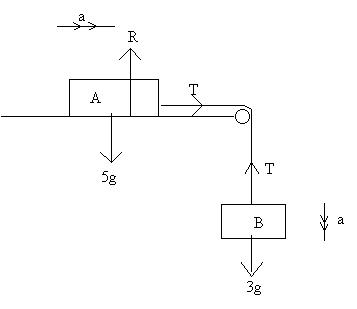## Using Newton's Laws

Newton's Laws of Motion can be used to work out forces and accelerations of bodies.

Solving Problems

When solving problems you should always draw a diagram. Mark on all of the forces and accelerations.

Example

A body of mass 5kg lies on a smooth horizontal table. It is connected by a light inextensible string, which passes over a smooth pulley at the edge of the table, to another body of mass 3kg which is hanging freely. The system is released from rest. Find the tension in the string and the acceleration.

• Since the string is inextensible and the two bodies are connected, they will both accelerate at the same rate. If the body hanging freely accelerates at a certain rate, the string will pull the other body so that it accelerates at the same rate.

• The tension in the string on both sides of the pulley will also be the same. This is because the pulley is smooth.

• Remember, W = mg. The mass of the first body is 5 kg and so its weight is 5g N. The mass of the second body is 3 kg and so its weight is 3g N.

• Remember to mark in the normal reaction force, a consequence of Newton's Third Law.Using Newton's Second Law on body A (horizontally):

(F = ma)
T = 5a     (1)

Using Newton's Second Law on body B (vertically):

3g - T = 3a    (2)

Solving (1) and (2) simultaneously:
3g - 5a = 3a
8a = 3g
a = 3g/8
T = 15g/8

Therefore the acceleration is 3.68 ms-2 (3sf) and the tension is 18.4 N (3sf)